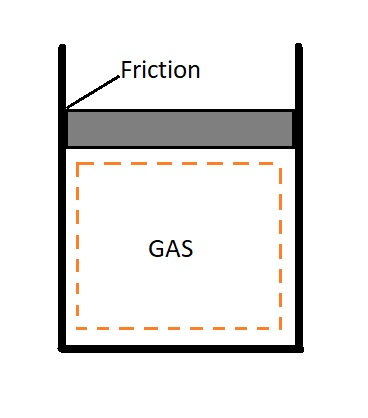# Irreversible vs. Reversible Process

As stated by the second law of thermodynamics, no heat engine can be 100% efficient.  However, what is the highest efficiency that a heat engine can possibly have?  To help answer this question we will characterize a reversible process, which is an idealized process.

### Reversible Process

So what is a reversible process?  In order for a process to be reversible it must be able to go back to its original state without leaving any trace on its surroundings.  This is the case for both the system and its surroundings. In order for this to occur, the net heat and net work exchange between the system and the its surrounding  must be zero.  Any process that cannot meet these guidelines is called an irreversible process.

It is, however, important to note that a system with an irreversible process can be returned to its initial state.  However, the fact that a system can be returned to its initial state does not mean that the process is reversible.  The reason for this is because the process must not have a net change on the surrounding environment.  If work is required to return the system to its initial state than the process is not reversible.

In reality, a reversible process cannot occur in nature.  The reason why is because its violates the second law of thermodynamics.  Instead, a reversible process is an idealized process.  As a result, it can be used to approximate how an actual device would behave.  However, it is important to realize that this process can never be achieved. In other words, all processes found in nature are irreversible.

Even though a reversible process cannot actually occur, there are two reason why they are of interest.  First, a reversible process is simple to analyze.  This is because the system will pass through a series of equilibrium states.  Secondly, a reversible process is the ideal model.  As a result, this ideal model can be compared to the actual model to determine  the theoretical limits of the actual model.

### Irreversibilities

Any factor that causes are process to be irreversible is called an irreversiblity.  In turn,  this includes friction, mixing of two fluids, electrical resistance, chemical reactions, unrestrained expansion, heat transfer across a finite temperature difference, and inelastic deformation of a solid, to name a few.  If any of these irrversibilities are present than the process is irreversible.

##### Friction

Friction is one of the most common irreversiblity that is associated with bodies that are in motion.  Frictional forces occur on the interfacing surfaces of two bodies that move against each other.  In turn, the frictional force causes energy to be lost as heat.  This energy loss is irreversible.

For example, let’s take a look at a piston.  The movement of a piston is a cyclic.  In other words, the piston will return to its starting position when its cycle is complete.  However, during the cycle the piston is typically in contact with the surface of a cylinder.  As the piston moves frictional force will develop.  Energy is required to overcome these frictional forces.  In turn, the energy turns into heat.  As a result, since friction is an irreversiblity this heat will not be converted back to the work energy required to run the piston.  Instead more work energy will continue to be converted to heat.  As a result, additional energy will need to be added to the system to keep the piston running. This will result in an irreversible process.Friction does not only involve two solid bodies.  In fact friction occurs between fluid and solids, as well as between layers of fluid moving at different velocities.  For example,  a considerable amount of power produced by a car engine is used to overcome drag forces between the air and the body of the car.  In turn, this energy will eventually raise the internal energy of the air.  It is also not possible to recover this energy, which in turn result in an irreversible process.

### Reversible Processes: Internal and External

When a process occurs, there will be interactions between a system and its surroundings.  In order for the process to be reversible, there must not be any irreversibilities associated with either.

For a process to be internally reversible, than there will be no irreversibilities within the boundaries of the system.  Hence, as a system proceeds through a series of equilibriums, it will pass through these same equilibriums as it returns to its initial state.   For example, a quasi-equilibrium process is an example of a reversible process.

In contrast, an external reversible process occurs when there are no irreversibilities outside of the system.  For example, if the outer surface of a system is at the same temperature as its surrounding than the heat transfer between the two would be externally reversible.

Finally, a total reversible process is both externally and internally reversible.  This means that there will be no friction, no nonquasi-equilibrium changes, no heat transfer due to finite temperature difference, or other dissipative effects.